# Three-dimensional space

For a broader, less mathematical treatment related to this topic, see Space.
"Three-dimensional" redirects here. For other uses, see 3D (disambiguation).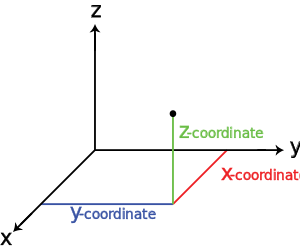A representation of a three-dimensional Cartesian coordinate system with the x-axis pointing towards the observer.

Three-dimensional space (also: 3-space or, rarely, tri-dimensional space) is a geometric setting in which three values (called parameters) are required to determine the position of an element (i.e., point). This is the informal meaning of the term dimension.

In physics and mathematics, a sequence of n numbers can be understood as a location in n-dimensional space. When n = 3, the set of all such locations is called three-dimensional Euclidean space. It is commonly represented by the symbol 3. This serves as a three-parameter model of the physical universe (that is, the spatial part, without considering time) in which all known matter exists. However, this space is only one example of a large variety of spaces in three dimensions called 3-manifolds. In this classical example, when the three values refer to measurements in different directions (coordinates), any three directions can be chosen, provided that vectors in these directions do not all lie in the same 2-space (plane). Furthermore, in this case, these three values can be labeled by any combination of three chosen from the terms width, height, depth, and breadth.

## In euclidean geometry

### Coordinate systems

Main article: Coordinate system

In mathematics, analytic geometry (also called Cartesian geometry) describes every point in three-dimensional space by means of three coordinates. Three coordinate axes are given, each perpendicular to the other two at the origin, the point at which they cross. They are usually labeled x, y, and z. Relative to these axes, the position of any point in three-dimensional space is given by an ordered triple of real numbers, each number giving the distance of that point from the origin measured along the given axis, which is equal to the distance of that point from the plane determined by the other two axes.

Other popular methods of describing the location of a point in three-dimensional space include cylindrical coordinates and spherical coordinates, though there are an infinite number of possible methods. See Euclidean space.

Below are images of the above-mentioned systems.

### Lines and planes

Two distinct points always determine a (straight) line. Three distinct points are either collinear or determine a unique plane. Four distinct points can either be collinear, coplanar or determine the entire space.

Two distinct lines can either intersect, be parallel or be skew. Two parallel lines, or two intersecting lines, lie in a unique plane, so skew lines are lines that do not meet and do not lie in a common plane.

Two distinct planes can either meet in a common line or are parallel (do not meet). Three distinct planes, no pair of which are parallel, can either meet in a common line, meet in a unique common point or have no point in common. In the last case, the three lines of intersection of each pair of planes are mutually parallel.

A line can lie in a given plane, intersect that plane in a unique point or be parallel to the plane. In the last case, there will be lines in the plane that are parallel to the given line.

A hyperplane is a subspace of one dimension less than the dimension of the full space. The hyperplanes of a three-dimensional space are the two-dimensional subspaces, that is, the planes. In terms of cartesian coordinates, the points of a hyperplane satisfy a single linear equation, so planes in this 3-space are described by linear equations. A line can be described by a pair of independent linear equations, each representing a plane having this line as a common intersection.

Varignon's theorem states that the midpoints of any quadrilateral in ℝ3 form a parallelogram, and so, are coplanar.

### Spheres and balls

Main article: Sphere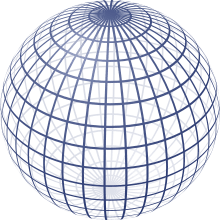A two-dimensional perspective projection of a sphere

A sphere in 3-space (also called a 2-sphere because it is a 2-dimensional object) consists of the set of all points in 3-space at a fixed distance r from a central point P. The solid enclosed by the sphere is called a ball (or, more precisely a 3-ball). The volume of the ball is given by.

Another type of sphere arises from a 4-ball, whose three-dimensional surface is the 3-sphere: points equidistant to the origin of the euclidean space 4. If a point has coordinates, P(x, y, z, w), then x2 + y2 + z2 + w2 = 1 characterizes those points on the unit 3-sphere centered at the origin.

### Polytopes

Main article: Polyhedron

In three dimensions, there are nine regular polytopes: the five convex Platonic solids and the four nonconvex Kepler-Poinsot polyhedra.

Regular polytopes in three dimensions
Class Platonic solids Kepler-Poinsot polyhedra
Symmetry Td Oh Ih
Coxeter group A3, [3,3] B3, [4,3] H3, [5,3]
Order 24 48 120
Regular
polyhedron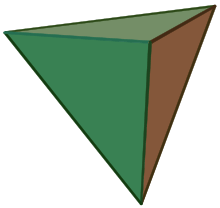{3,3}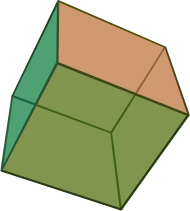{4,3}{3,4}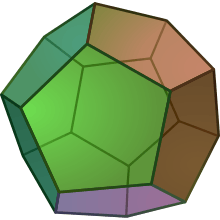{5,3}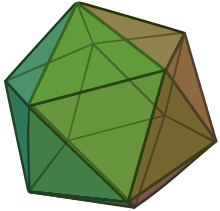{3,5}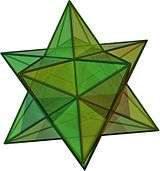{5/2,5}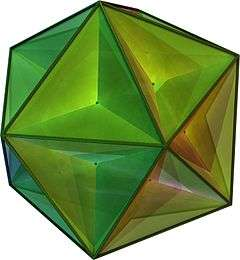{5,5/2}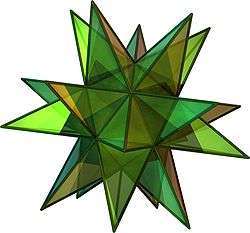{5/2,3}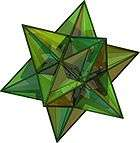{3,5/2}

### Surfaces of revolution

Main article: Surface of revolution

A surface generated by revolving a plane curve about a fixed line in its plane as an axis is called a surface of revolution. The plane curve is called the generatrix of the surface. A section of the surface, made by intersecting the surface with a plane that is perpendicular (orthogonal) to the axis, is a circle.

Simple examples occur when the generatrix is a line. If the generatrix line intersects the axis line, the surface of revolution is a right circular cone with vertex (apex) the point of intersection. However, if the generatrix and axis are parallel, the surface of revolution is a circular cylinder.

In analogy with the conic sections, the set of points whose cartesian coordinates satisfy the general equation of the second degree, namely,where A, B, C, F, G, H, J, K, L and M are real numbers and not all of A, B, C, F, G and H are zero is called a quadric surface.

There are six types of non-degenerate quadric surfaces:

The degenerate quadric surfaces are the empty set, a single point, a single line, a single plane, a pair of planes or a quadratic cylinder (a surface consisting of a non-degenerate conic section in a plane π and all the lines of 3 through that conic that are normal to π). Elliptic cones are sometimes considered to be degenerate quadric surfaces as well.

Both the hyperboloid of one sheet and the hyperbolic paraboloid are ruled surfaces, meaning that they can be made up from a family of straight lines. In fact, each has two families of generating lines, the members of each family are disjoint and each member one family intersects, with just one exception, every member of the other family.

## In linear algebra

Another way of viewing three-dimensional space is found in linear algebra, where the idea of independence is crucial. Space has three dimensions because the length of a box is independent of its width or breadth. In the technical language of linear algebra, space is three-dimensional because every point in space can be described by a linear combination of three independent vectors.

### Dot product, angle, and length

Main article: Dot product

A vector can be pictured as an arrow. Its magnitude is its length, and its direction is the direction the arrow points. A vector in 3 can be represented by an ordered triple of real numbers. These numbers are called the components of the vector.

The dot product of two vectors A = [A1, A2, A3] and B = [B1, B2, B3] is defined as:The magnitude of a vector A is denoted by ||A||. The dot product of a vector A = [A1, A2, A3] with itself iswhich givesthe formula for the Euclidean length of the vector.

Without reference to the components of the vectors, the dot product of two non-zero Euclidean vectors A and B is given bywhere θ is the angle between A and B.

### Cross product

Main article: Cross product

The cross product or vector product is a binary operation on two vectors in three-dimensional space and is denoted by the symbol ×. The cross product a × b of the vectors a and b is a vector that is perpendicular to both and therefore normal to the plane containing them. It has many applications in mathematics, physics, and engineering.

The space and product form an algebra over a field, which is neither commutative nor associative, but is a Lie algebra with the cross product being the Lie bracket.

One can in n dimensions take the product of n − 1 vectors to produce a vector perpendicular to all of them. But if the product is limited to non-trivial binary products with vector results, it exists only in three and seven dimensions.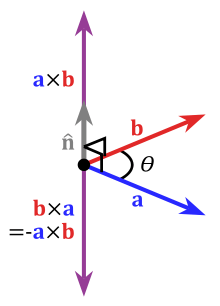The cross-product in respect to a right-handed coordinate system

## In calculus

Main article: vector calculus

In a rectangular coordinate system, the gradient is given byThe divergence of a continuously differentiable vector field F = U i + V j + W k is equal to the scalar-valued function:Expanded in Cartesian coordinates (see Del in cylindrical and spherical coordinates for spherical and cylindrical coordinate representations), the curl ∇ × F is, for F composed of [Fx, Fy, Fz]:where i, j, and k are the unit vectors for the x-, y-, and z-axes, respectively. This expands as follows:### Line integrals, surface integrals, and volume integrals

For some scalar field f : URnR, the line integral along a piecewise smooth curve CU is defined aswhere r: [a, b] → C is an arbitrary bijective parametrization of the curve C such that r(a) and r(b) give the endpoints of C and.

For a vector field F : URnRn, the line integral along a piecewise smooth curve CU, in the direction of r, is defined aswhere · is the dot product and r: [a, b] → C is a bijective parametrization of the curve C such that r(a) and r(b) give the endpoints of C.

A surface integral is a generalization of multiple integrals to integration over surfaces. It can be thought of as the double integral analog of the line integral. To find an explicit formula for the surface integral, we need to parameterize the surface of interest, S, by considering a system of curvilinear coordinates on S, like the latitude and longitude on a sphere. Let such a parameterization be x(s, t), where (s, t) varies in some region T in the plane. Then, the surface integral is given bywhere the expression between bars on the right-hand side is the magnitude of the cross product of the partial derivatives of x(s, t), and is known as the surface element. Given a vector field v on S, that is a function that assigns to each x in S a vector v(x), the surface integral can be defined component-wise according to the definition of the surface integral of a scalar field; the result is a vector.

A volume integral refers to an integral over a 3-dimensional domain.

It can also mean a triple integral within a region D in R3 of a functionand is usually written as:### Fundamental theorem of line integrals

The fundamental theorem of line integrals, says that a line integral through a gradient field can be evaluated by evaluating the original scalar field at the endpoints of the curve.

Let. Then### Stokes' theorem

Main article: Stokes' theorem

Stokes' theorem relates the surface integral of the curl of a vector field F over a surface Σ in Euclidean three-space to the line integral of the vector field over its boundary ∂Σ:### Divergence theorem

Main article: Divergence theorem

Suppose V is a subset of(in the case of n = 3, V represents a volume in 3D space) which is compact and has a piecewise smooth boundary S (also indicated with V = S). If F is a continuously differentiable vector field defined on a neighborhood of V, then the divergence theorem says: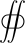The left side is a volume integral over the volume V, the right side is the surface integral over the boundary of the volume V. The closed manifold V is quite generally the boundary of V oriented by outward-pointing normals, and n is the outward pointing unit normal field of the boundary V. (dS may be used as a shorthand for ndS.)

## In topology

Three-dimensional space has a number of topological properties that distinguish it from spaces of other dimension numbers. For example, at least three dimensions are required to tie a knot in a piece of string.

With the space, the topologists locally model all other 3-manifolds.

1. Brannan, Esplen & Gray 1999, pp. 34–5
2. Brannan, Esplen & Gray 1999, pp. 41–2
3. Anton 1994, p. 133
4. Anton 1994, p. 131
5. WS Massey (1983). "Cross products of vectors in higher dimensional Euclidean spaces". The American Mathematical Monthly. 90 (10): 697–701. doi:10.2307/2323537. JSTOR 2323537. If one requires only three basic properties of the cross product ... it turns out that a cross product of vectors exists only in 3-dimensional and 7-dimensional Euclidean space.
6. Arfken, p. 43.
7. M. R. Spiegel; S. Lipschutz; D. Spellman (2009). Vector Analysis. Schaum’s Outlines (2nd ed.). USA: McGraw Hill. ISBN 978-0-07-161545-7.
8. Rolfsen, Dale (1976). Knots and Links. Berkeley, California: Publish or Perish. ISBN 0-914098-16-0.
• Anton, Howard (1994), Elementary Linear Algebra (7th ed.), John Wiley & Sons, ISBN 978-0-471-58742-2
• Arfken, George B. and Hans J. Weber. Mathematical Methods For Physicists, Academic Press; 6 edition (June 21, 2005). ISBN 978-0-12-059876-2.
• Brannan, David A.; Esplen, Matthew F.; Gray, Jeremy J. (1999), Geometry, Cambridge University Press, ISBN 978-0-521-59787-6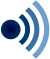Wikiquote has quotations related to: Three-dimensional spaceWikimedia Commons has media related to 3D.
•The dictionary definition of three-dimensional at Wiktionary